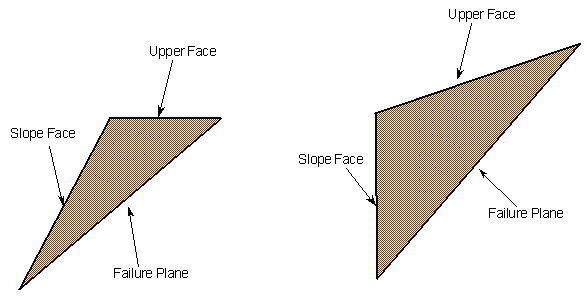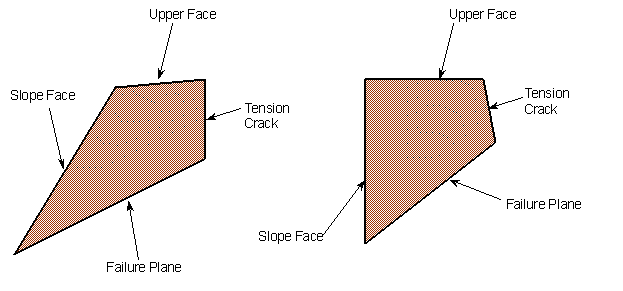The Rocscience International Conference 2021 Proceedings are now available. Read Now

# Geometry Validation

## Valid Geometry

RocPlane always checks if the model geometry is valid before proceeding to calculate a Factor of Safety for a given wedge. RocPlane checks whether the specified Slope Face, Upper Face, and Failure Plane form a legitimate sliding block or wedge. The figures below provide examples of valid sliding blocks.Examples of valid sliding block geometries, when no Tension Crack is involved

## Valid Geometry with Tension Crack

In addition, RocPlane examines how the Tension Crack intersects the other planes, accepting only those cases where it truncates the wedge in a kinematically admissible manner. If a Tension Crack is defined and a Bench Width specified, the Bench Width cannot define a wedge that is smaller than the one defined by the distance of the Tension Crack from the crest. An error message is displayed if this is the case. Examples of valid sliding block geometries with Tension Cracks are provided in the figures below.Examples of valid wedge geometries involving Tension Cracks

## Error Messages

If the Analysis Type = DETERMINISTIC

• You receive an error message if there is a problem with your input geometry.

If the Analysis Type = PROBABILISTIC

• Validation is first performed on the mean Input Data. If the mean orientation data does not form a valid wedge, the entire Probabilistic analysis is aborted, and you receive an error message. If the mean wedge is valid but invalid wedges are generated during the statistical sampling, results are discarded but the analysis is allowed to proceed. You can see the Number of Valid Wedges for a Probabilistic analysis in the Info Viewer.